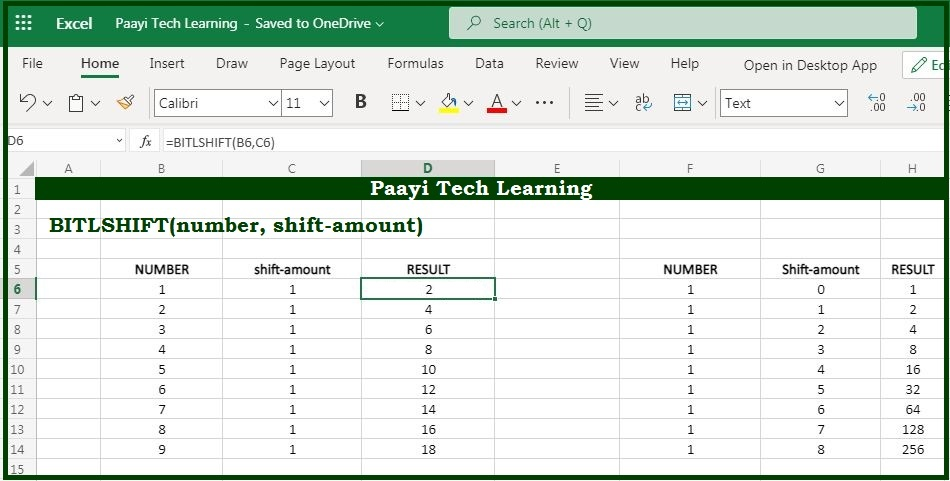# Learn How to Use Microsoft Excel BITLSHIFT Function

Written by | 0 Comments | 686 Views

In this article, you will learn how to use the Microsoft Excel BITLSHIFT function and its prime function in Microsoft Excel. You will also get to know the Microsoft Excel BITLSHIFT function return value and syntax with the help of some examples.

## Microsoft Excel BITLSHIFT Function

The main function of the Microsoft Excel BITLSHIFT function is used to return a number shifted left by some number of bits. So with the help of the BITLSHIFT function, you can able to shift a number by a specified number of bits. You can able to effectively double, half the number specified number of times.

### Return Value of BITLSHIFT Function

The return value will be the decimal number.

### Syntax of BITLSHIFT Function

=BITLSHIFT(number, shift-amount)

Where the arguments:

number: This is the number to be shifted as per bit.

shift-amount: It is the amount of bits to shift, in case negative shifts bits to the right instead.

## How to Use Microsoft Excel BITLSHIFT Function?The shift_amount can be negative or positive and if a negative number is provided, the bits are shifted to the right. For any bit shift that results in an integer overflow, where the result is greater than 2^48-1, the BITLSHIFT function will return the #NUM! error.